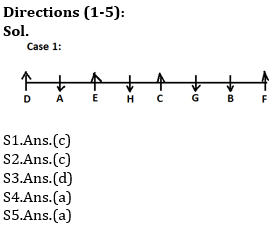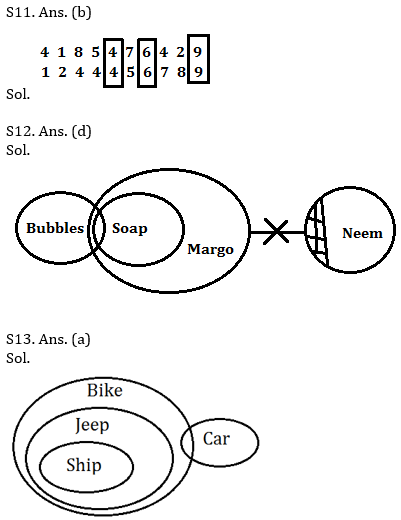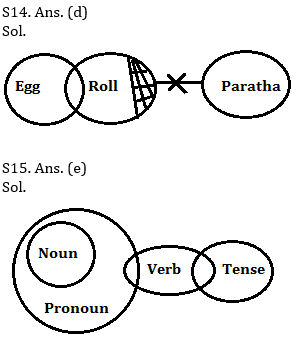Latest Banking jobs   »   Reasoning Ability Quiz For IBPS Clerk/NIACL...

# Reasoning Ability Quiz For IBPS Clerk/NIACL AO Prelims 2021- 6th September

Directions (1-5): Study the following information carefully and answer the questions given below:
Eight persons A, B, C, D, E, F, G and H are sitting in a row. Four of them are facing towards north while four of them are facing towards south. No two person sits adjacent to each other according to the English alphabet i.e. (B does not sits adjacent to A and C).
C sits third to the left of A. Only two person sits between C and F. A does not sits at the end of the row. E sits 4th to the right of B. H sits third to the right of D and faces south. G and H faces same direction. Immediate neighbour of H faces same direction. F faces north direction.

Q1. Who among the following pair are facing same direction?
(a) All of them are facing same direction
(b) A, D
(c) A, B
(d) D, H
(e) A, F

Q2. Who among the following person sits immediate right of A?
(a) G
(b) A
(c) D
(d) No one
(e) B

Q3. What is the position of H with respect to F?
(a) Fourth to the right
(b) Immediate left
(c) Second to the right
(d) Fourth to the left
(e) Second to the left

Q4. How many persons are sitting between G and D?
(a) Four
(b) Two
(c) No one
(d) Three
(e) Six

Q5. Who among the following person are immediate neighbors of B?
(a) F, G
(b) A, F
(c) F, H
(d) D, C
(e) None of these

Directions (6-10): Study the following arrangement carefully and answer the questions given below:
2 3 5 7 1 2 6 4 5 7 1 9 8 5 2 4 1 4 5 8 2 6 3 7 6 9 4 1 5 6 7

Q6. Which of the following digit will be seventh to the right of eleventh from the left end?
(a) 2
(b) 4
(c) 5
(d) 7
(e) None of these

Q7. How many 2’s are there in the series which are followed by a number, which is less than or equal to 5?
(a) One
(b) None
(c) three
(d) Two
(e) More than three

Q8. What will be the addition of fourth and twelfth digit from the right end and eleventh digit from the left end?
(a) 12
(b) 11
(c) 13
(d) 10
(e) None of these

Q9. If all the 2’s and 4’s are removed from the series, then which of the following will be fifth to the right of twelfth digit from the left end?
(a) 7
(b) 3
(c) 8
(d) 6
(e) None of these

Q10. How many 7’s are there in the series which are immediately preceded by an odd number and immediately followed by a number?
(a) One
(b) None
(c) three
(d) Two
(e) More than three

Q11. How many such numerals are there in the number ‘4185476429’ which will remain at the same position when arranged in ascending order from left to right?
(a) None
(b) Three
(c) More than three
(d) Two
(e) None of these

Directions (12-15): In each of the questions below are given some statements followed by some conclusions. You have to take the given statements to be true even if they seem to be at variance with commonly known facts. Read all the conclusions and then decide which of the given conclusions logically follows from the given statements disregarding commonly known facts.
(a) If only conclusion I follows.
(b) If only conclusion II follows.
(c) If either conclusion I or II follows.
(d) If neither conclusion I nor II follows.
(e) If both conclusions I and II follow.

Q12. Statements: Only a few Bubbles are Soap. All Soap are Margo. Some Neem are not Margo.
Conclusions:
I. Some Bubbles are not Neem.
II. Some Soap are not Bubbles.

Q13. Statements: All Ship are Jeep. All Jeep are Bike. Some Car are Bike.
Conclusions:
I.
Some Ship can be Car.
II. All Car are Bike.

Q14. Statements: Some Egg are Roll. Some Roll are not Paratha.
Conclusions:
I.
All Egg can never be Paratha.
II. Some Paratha are not Roll.

Q15. Statements: All Noun are Pronoun. Some Pronoun are Verb. Some Verb are Tense.
Conclusions:
I.
Some Noun can be Verb.
II. Some Tense being Pronoun is a possibility.

SolutionsS6.Ans.(b)
Sol.
The element seventh to the right of eleventh from the left end is (11+7)= 18th from the left end i.e. is ‘4’.

S7.Ans.(d)
Sol. There are only two 2’s in the series which are followed by a number, which is less than or equal to 5 i.e. ‘2 3’ and ‘2 4’.

S8.Ans.(d)
Sol.
The addition of fourth and twelfth element from the right = (1+8)= 9.
The eleventh digit from the left end is = 1
Hence, addition is = (9+1)=10

S9.Ans.(a)
Sol.
After removing 2’s and 4’s is
3 5 7 1 6 5 7 1 9 8 5 1 5 8 6 3 7 6 9 1 5 6 7
Then, the element fifth to the right of twelfth from the left end is ‘7’.

S10.Ans.(c)
Sol.
There are only three 7’s which are immediately by an odd number and immediately followed by a number i.e. ‘5 7 1’, ‘5 7 1’ and ‘3 7 6’.Click Here to Register for Bank Exams 2021 Preparation Material#### Congratulations!Download Hindu Review of October 2021: Free PDF